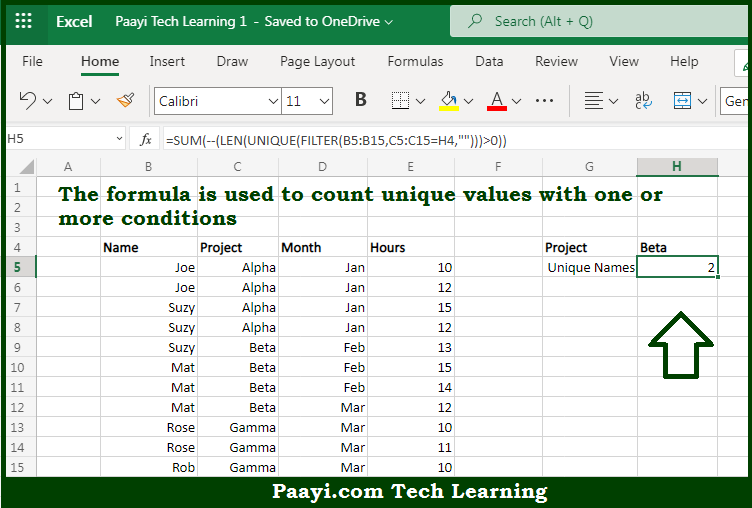# Learn How to Count Unique Values With Criteria in Microsoft Excel

Written by | 0 Comments | 559 Views

In this article, you will learn how to evaluate things in Dynamic Arrays with formulas in Microsoft Excel using a single/combination(s) of functions. You will also know How to Count Unique Values With Criteria and see the generic formula.

How to Count Unique Values With Criteria in Microsoft Excel

The main purpose of this formula is to count unique values with one or more conditions. Here we will learn how to count unique values with criteria from the given data set in the workbook in Microsoft Excel. That implies, with the help of a formula based on the UNIQUE and FILTER function you can able to count unique values with one or more conditions in a set of data. So, with the help of this formula, you can able to count unique values with criteria from the given data set in the workbook in Microsoft Excel.

General Formula to Count Unique Values With Criteria

=SUM(--(LEN(UNIQUE(FILTER(range,criteria,"")))>0))

The Explanation for the Count Unique Values With CriteriaSo we know that with the help of the given formula above you can able to count unique values with one or more conditions. Here we will learn how to count unique values with criteria from the given data set in the workbook in Microsoft Excel. As we know that here the formula uses the UNIQUE function to extract unique values, and the FILTER function is used to apply criteria. When working from the inside out, the FILTER function is used to apply criteria and extract only names that are associated with the given project. So, with the help of this formula, you can able to count unique values with one or more conditions. Here we will learn how to count unique values with criteria from the given data set in the workbook in Microsoft Excel.